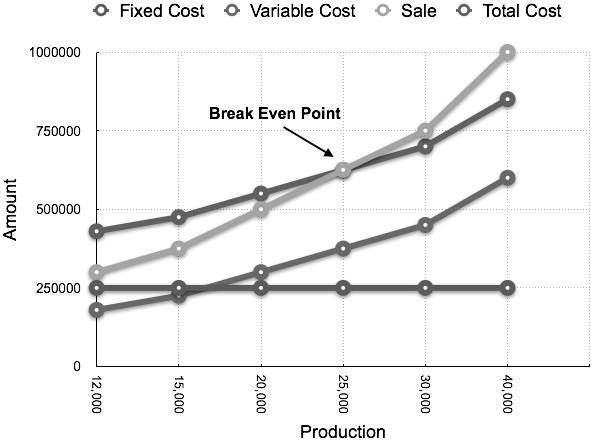### Cost Accounting - CVP Analysis

Cost-Volume-Profit (CVP) Analysis is also known as Break–Even Analysis. Every business organization works to maximize its profits. With the help of CVP analysis, the management studies the co-relation of profit and the level of production.
CVP analysis is concerned with the level of activity where total sales equals the total cost and it is called as the break-even point. In other words, we study the sales value, cost and profit at different levels of production. CVP analysis highlights the relationship between the cost, the sales value, and the profit.

## Assumptions

Let us go through the assumptions for CVP analysis:
• Variable costs remain variable and fixed costs remain static at every level of production.
• Sales volume does not affect the selling price of the product. We can assume the selling price as constant.
• At all level of sales, the volume, material, and labor costs remain constant.
• Efficiency and productivity remains unchanged at all the levels of sales volume.
• The sales-mix at all level of sales remains constant in a multi-product situation.
• The relevant factor which affects the cost and revenue is volume only.
• The volume of sales is equal to the volume of production.

## Marginal Cost Equation

Equations for elements of cost are as follows:
```Sales = Variable costs + Fixed Expenses ± Profit /Loss

Or

Sales – Variable Cost = Fixed Expenses ± Profit /Loss

Or

Sales – Variable Cost = Contribution
```
It is necessary to understand the following four concepts, their calculations, and applications to know the mathematical relation between cost, volume, and profit:
• Contribution
• Profit Volume Ratio (P/V Ratio or Contribution/Sales (C/S))
• Break-Even Point
• Margin of Safety

### Contribution

```Contribution = Sales – Marginal Cost
```
We have already discussed contribution in Marginal Costing topic above.

### Profit-Volume Ratio

Profit / Volume (P/V) ratio is calculated while studying the profitability of operations of a business and to establish a relation between Sales and Contribution. It is one of the most important ratios, calculated as under:
PV Ratio =
ContributionSales
Fixed Expenses+ProfitSales
Sales−Variable CostSales
Change in profits of ContributionsChange in Sales
The P/V Ratio shares a direct relation with profits. Higher the P/V ratio, more the profit and vice-a-versa.

### Break-Even Point

When the total cost of executing business equals to the total sales, it is called break-even point. Contribution equals to the fixed cost at this point. Here is a formula to calculate break-even point:
B.E.P (in units) =
Total Fixed ExpensesSelling Price per Unit − Marginal Cost per Unit
Total Fixed ExpensesContribution per Unit
Break-even point based on total sales:
Fixed CostPV Ratio
Calculation of output or sales value at which a desired profit is earned:
Fixed Expenses + Desired ProfitSelling Price per Unit − Marginal Cost per Unit
Fixed Expenses + Desired ProfitContribution per Unit

#### COMPOSITE BREAK EVEN POINT

A company may have different production units, where they may produce the same product. In this case, the combined fixed cost of each productions unit and the combined total sales are taken into consideration to find out BEP.
• Constant Product - Mix Approach In this approach, the ratio is constant for the products of all production units.
• Variable Product - Mix Approach In this approach, the preference of products is based on bigger ratio.

### Margin of Safety

Excess of sale at BEP is known as margin of safety. Therefore,
```Margin of safety = Actual Sales − Sales at BEP
```
Margin of safety may be calculated with the help of the following formula:
Margin of Safety =
ProfitPV Ratio
ProfitContribution per Unit

## Break-Even Chart

Break-Even Chart is the most useful graphical representation of marginal costing. It converts accounting data to a useful readable report. Estimated profits, losses, and costs can be determined at different levels of production. Let us take an example.

### Example

Calculate break-even point and draw the break-even chart from the following data:
```Fixed Cost    = Rs 2,50,000
Variable Cost = Rs 15 per unit
Selling Price = Rs 25 per unit
Production level in units 12,000, 15,000, 20,000, 25,000, 30,000, and 40,000.
```
Solution:
B.E.P =
Fixed CostContribution per unit
Rs 2,50,000Rs 10 × (Rs 25 - Rs 15)
= 25,000 units
At production level of 25,000 units, the total cost will be Rs 6,25,000.
(Calculated as (25000 × 14) + 2,50000)
 Statement showing Profit & Margin of safety at different level of production Break Even Sale = Rs 6,25,000 (25,000 x 25) Production (In Units) Total Sale (In Rs) Total Cost (In Rs) Profit (Sales - Cost) (In Rs) Margin of safety (Profit/Contribution per unit) (In Units) 12000 3,00,000 4,30,000 -1,30,000 15000 3,75,000 4,75,000 -1,00,000 20000 5,00,000 5,50,000 -50,000 25000 6,25,000 6,25,000 (B.E.P) (B.E.P) 30000 7,50,000 7,00,000 50,000 5,000 40000 10,00,000 8,50,000 1,50,000 15,000
The corresponding chart plotted as production against amount appears as follows:1.Ogymcucaezo Patrick Burrell https://wakelet.com/wake/PKcvki-hvvusf2SZuB0FU
recalnide

2.click
click
planerstordis

3.Vsubsferin-bi_1979 Nathan Albright Bootstrap Studio
The Bat!
Bootstrap Studio
profdecsystro